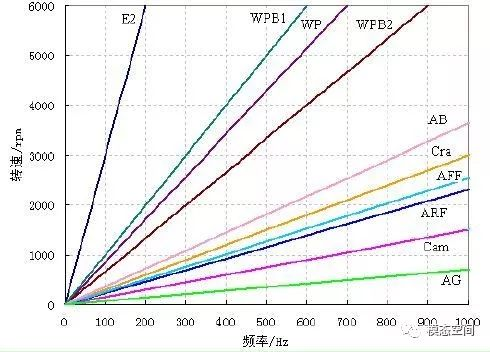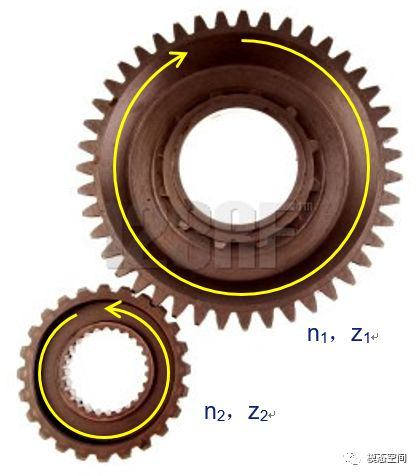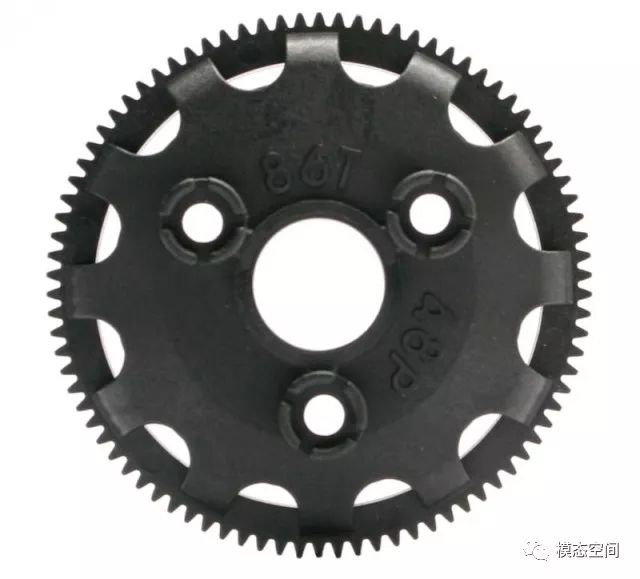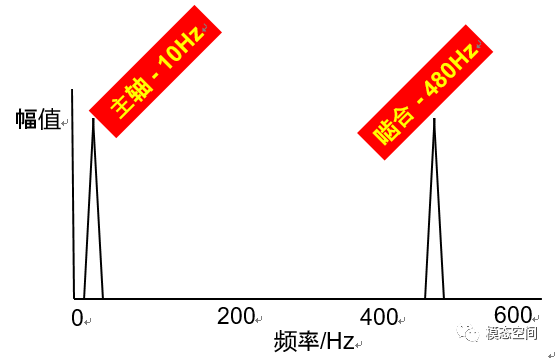# 齿轮的特征阶次E2：动机二阶激励； Cam：凸轮轴正时齿轮； Cra：曲轴正时齿轮  WP：水泵叶片； WPB1：水泵轴承滚珠； WPB2：水泵轴承滚柱；A：发电机； AFF：发电机前风扇； ARF：发电机后风扇；AB：发电机轴承； AG：发电机线槽

1

i12=-n1/n2=-z2/z1V2=d1ω1/2

i=n1/nk=各对齿轮传动比的连乘积

i1k=(-1)m所有从动轮齿数的连乘积/所有主动轮齿数的连乘积

23

a= z1/z2

 构件 转速 相对转速 齿数 数量 齿圈 n1 n1-nH z1 1 太阳轮 n2 n2-nH z2 1 行星轮 n3 n3-nH z3 n 行星架 nH 0 1

(n2-nH)z2=nHz1

i=n2/nH= (z1+z2)/z2=1+a

(n1-nH)z1=nHz2

i= nH/n1=z1/(z1+z2)=a/(1+a)

i= n2/n1=z1/z2=a

 No. 固定 主动轮 从动轮 传动比 1 齿圈 太阳轮 行星架 1+a 2 太阳轮 行星架 齿圈 a/(1+a) 3 行星架 太阳轮 齿圈 a 4 联锁任意两个构件 1
4

 No. 固定 主动轮 从动轮 啮合阶次 1 齿圈 太阳轮 行星架 z1/(1+a) 2 太阳轮 行星架 齿圈 z2 3 行星架 太阳轮 齿圈 z2